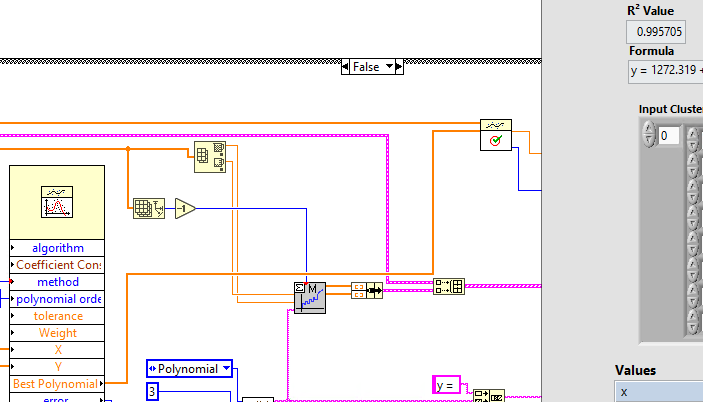# LabVIEW

cancel
Showing results for
Did you mean:

Solved!
Go to solution

## Goodness of Fit VI disagrees with Excel R^2 value

Hi,

I have a VI with graphing functionality of some values.

I want to display the formula and R2 value of the trendline. The trendline looks right and the formula pretty much exactly agrees with excel, however the R^2 value is way (compared to excel).

I'm not great at statistics, etc. so don't know which value is more accurate (my guess is excel). I'm guessing the discrepancy is to do with the number of points on the line that the "goodness of fit" VI is sampling, compared to perhaps a different number on Excel, but I have no idea.

My question: How do I get my LabView R2 to agree with the Excel R2?

Many thanks,

Charlie

Message 1 of 5
(410 Views)

## Re: Goodness of Fit VI disagrees with Excel R^2 value

@charliecameron00 wrote:

Hi,

I have a VI with graphing functionality of some values.

I want to display the formula and R2 value of the trendline. The trendline looks right and the formula pretty much exactly agrees with excel, however the R^2 value is way (compared to excel).

I'm not great at statistics, etc. so don't know which value is more accurate (my guess is excel). I'm guessing the discrepancy is to do with the number of points on the line that the "goodness of fit" VI is sampling, compared to perhaps a different number on Excel, but I have no idea.

My question: How do I get my LabView R2 to agree with the Excel R2?

Many thanks,

Charlie

You won't get them to agree.  Frankly, Excel does not treat floating point operations exactly as IEEE 754 requires for all floating point operations (because, it is a spreadsheet and optimized for financial data.)  LabVIEW DOES implement the entire IEEE 754 standard.  Therefore, when you perform rigorous statistical analysis in Excel there is a natural difference from any calculation performed with exact adherence to IEEE 754.

"Should be" isn't "Is" -Jay
Message 2 of 5
(360 Views)

## Re: Goodness of Fit VI disagrees with Excel R^2 value

@charliecameron00 wrote:

I'm not great at statistics, etc. so don't know which value is more accurate (my guess is excel). I'm guessing the discrepancy is to do with the number of points on the line that the "goodness of fit" VI is sampling, compared to perhaps a different number on Excel, but I have no idea.

Hi, Charlie.

You might not be "great at statistics", but you should at least know what R² is.  Here's a suggestion -- be an Engineer/scientist, and "do an experiment".  Take three of your points and ask Excel and LabVIEW to fit a quadratic (which seems to be your model).  They should both give you the same coefficients, and an R² of 1.  [Do you know why R² is 1 in this case?].  Add another point, and repeat the Experiment.

It would be of interest to some of us (to me, for example) if you attach an Excel Workbook with the 3-point and 4-point Experiments, showing the 3 (or 4) data points, Excel's coefficients and R², and LabVIEW's coefficients and R².

Bob Schor

Message 3 of 5
(352 Views)
Solution
Accepted by topic author charliecameron00

## Re: Goodness of Fit VI disagrees with Excel R^2 value

If you connect the "Goodness of fit" VI's "Best fit" input to the "Best polynomial" output of the General Polynomial Fit VI (instead of the output of the "Evaluate f(x)" VI) then you get an exact R² match.Message 4 of 5
(334 Views)

## Re: Goodness of Fit VI disagrees with Excel R^2 value

You won't get them to agree.  Frankly, Excel does not treat floating point operations exactly as IEEE 754 requires for all floating point operations (because, it is a spreadsheet and optimized for financial data.)  LabVIEW DOES implement the entire IEEE 754 standard.  Therefore, when you perform rigorous statistical analysis in Excel there is a natural difference from any calculation performed with exact adherence to IEEE 754.

That makes a lot of sense, thanks for clearing up. It annoys me how often excel cuts corners (without being clear about it) 😅

Message 5 of 5
(270 Views)